Courses

# Test: Thin Cylinder Level - 2

## 10 Questions MCQ Test Machine Design | Test: Thin Cylinder Level - 2

Description
This mock test of Test: Thin Cylinder Level - 2 for Mechanical Engineering helps you for every Mechanical Engineering entrance exam. This contains 10 Multiple Choice Questions for Mechanical Engineering Test: Thin Cylinder Level - 2 (mcq) to study with solutions a complete question bank. The solved questions answers in this Test: Thin Cylinder Level - 2 quiz give you a good mix of easy questions and tough questions. Mechanical Engineering students definitely take this Test: Thin Cylinder Level - 2 exercise for a better result in the exam. You can find other Test: Thin Cylinder Level - 2 extra questions, long questions & short questions for Mechanical Engineering on EduRev as well by searching above.
QUESTION: 1

### A thin cylindrical vessel closed at both ends is subjected to fluid pressure resulting in a hoop stress of 24 N/mm2. The maximum shear stress in the cylinder is

Solution: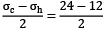= 6

QUESTION: 2

### A 600 mm diameter pipe contains a fluid at a pressure of 3 N/mm2. If the safe stress in tension is 75 N/mm2, the minimum thickness of pipe is equal to

Solution: Diameter, d = 600mm Pressure,

P = 3 N/mm2 Safe

streee = 75 N/mm2 Minimum thickness, t =?

75 =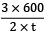t = 12mm

QUESTION: 3

### In a thin cylinder, hoop stress is 48 N/mm2. The max. Shear stress developed in the pipe material is

Solution: σh = 48 N/mm2 , σlongitudinal = σl = 24 N/mm2

Max shear stress,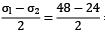=12N/mm2

QUESTION: 4

A cylinder of internal diameter 2.5m and of thickness 5cm contains a gas. If the tensile stress in the material is not to exceed 80 N/mm2, the internal pressure of the gas should be

Solution:

80 =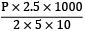P= 3.2 N/mm2

QUESTION: 5

A pressure vessel in the form of a thin cylinder of 1 m diameter and 1mm plate thickness is subjected to an internal fluid pressure of 0.2MPa. The maximum shear stress in the material is

Solution: d = 1 m = 103 mm t = 1 mm P = 0.2 MPa

τmax =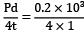= 50 MPa

QUESTION: 6

A cylinder of thickness 1.5cm has to withstand maximum internal pressure of 1.5 N/mm2. If the ultimate tensile stress in the material of the cylinder is 300 N/mm2, factor of safety 3.0 and joint efficiency 80%, the diameter of the cylinder is

Solution:

300/3 =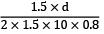d = 1600 mm = 160 cm

QUESTION: 7

In case of thin walled cylinder the ratio of hoop strain to Volumetric strain is

Solution: Hoop strain,

εh =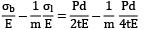Longitudinal strain,

εl =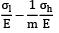=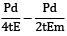Volumetric strain,

εv = εl + 2εb

=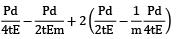=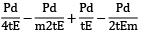εv=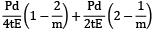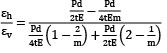=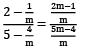=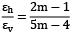QUESTION: 8

A thin cylindrical shell of diameter ‘d’, length ‘l’ and thickness ‘t’ is subjected to an internal pressure ‘p’. What is the ratio of longitudinal strain to hoop strain in terms of Poisson’s ratio (1/m)?

Solution: For a thin cylinder, longitudinal strain,

εl =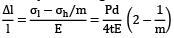Now,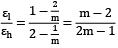QUESTION: 9

A cylindrical steel pressure vessel 400mm in diameter with a wall thickness of 20mm is subjected to an internal pressure of 4.5 MPa. Calculate the circumferential stresses (σx ) and longitudinal stresses (σy ) in the steel vessel.

Solution: Diameter = 400mm t = 20mm

p = 4.5 MPa

Circumferential stresses,

σx =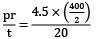σx = 45 MPa

Longitudinal stresses,

σy =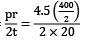σy = 22.5 MPa

QUESTION: 10

A water tank, 7000 mm in diameter, is made from steel plates that are 15 mm. thick. Find the maximum height to which the tank may be filled if the circumferential stress is limited to 40 MPa. The specific weight of water is 9800 N/m3

Solution: Diameter = 7000 mm t = 15mm Pressure of water = rh = 9800 h N/m2

Maximum circumferential stress,

=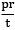= 40 MPa

40 =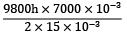h = 8.75m × 2 = 17.5m